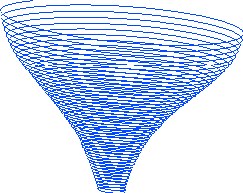Ordinary Differential Equations using MATLAB by David Arnold & John C. Polking

This is a manual for using MATLAB in a course on Ordinary Differential Equations. It can be used as a supplement of almost any textbook.

The manual completely describes two special MATLAB routines. `DFIELD5` is a very easy to use routine which takes a user defined first order differential equation, and plots its direction field. It also allows the user to plot solution curves.

`PPLANE5` plots vector fields for planar autonomous systems. It allows the user to plot solution curves in the phase plane. It also enables the user to plot these solutions in a variety of plots versus the independent variable. There are a number of advanced features, such as finding equilibrium points, displaying linearizations, and plotting separatrices.

The built-in MATLAB commands for solving ODEs are completely described in their most elementary usages, including the new ODE suite in version 5 of MATLAB. There is a treatment of linear algebra using MATLAB that is sufficient for understanding first order systems with constant coefficients.

The second edition was published by Prentice Hall in the Summer of 1999. Information about ordering the manual can be obtained from them. The ISBN number is 0-13-011381-6. The Table of Contents and Preface are available in either postscript or Adobe Acrobat formats.

There are several different versions of `DFIELD` and `PPLANE` which have been developed over the years for use with different versions of MATLAB. A description and instructions for downloading them are available.

John C. Polking < polking@rice.edu>